Next: Lie Algebras Up: Lie Groups and Quantum Mechanics Previous: A Rough Road-map

# Lie Groups

A Lie matrix group is a continuous subgroup of the group of all non-singularmatrices over a field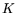, whereis either R or C. Continuous'' really is a shorthand for saying that the Lie group is a manifold. The rough idea is that the components of a matrix in the group can vary smoothly; thus, concepts like differentiable function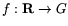'' should make sense. I'll just say Lie group'' for Lie matrix group, though many mathematicians would groan at this.

Example:is the group of all orthogonalmatrices, i.e. all matriceswith real components such that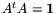. This is just the group of all isometries of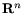which leave the origin fixed. (Standard calculation: let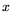be a column vector. Then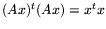, i.e., the norm ofequals the norm of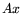.) Note also that the equations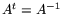and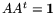follow from.

If, then we have immediately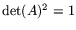, i.e.,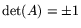.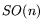is the subgroup of all matrices inwith determinant 1. Fact:is connected, and is in fact the connected component of  1in. I will focus initially on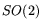and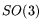. These are, colloquially, the groups of rotations in 2-space and 3-space.is the group of reflections and rotations.

Digression: the well-known puzzle, why do mirrors reverse left and right, but not up and down?'' is resolved mathematically by pointing out that a mirror perpendicular to the y-axis performs the reflection: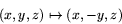i.e., corresponds to the following matrix in: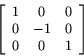For some psychological reason, people tend to think of this as the composition of a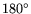rotation about the z-axis followed by a reflection in a plane perpendicular to the x-axis: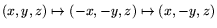. This makes it seem that the mirror is treating the x and z axes differently (left/right vs. updown), though it really isn't. End of digression.

Example:, rotations in 2-space. Since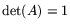, it is easy to write down the components of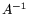. Equating these to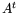, we see thathas the form: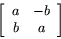with the constraint that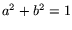. We can set up a one-one correspondence between this matrix and the complex number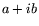on the unit circle. This is a Lie group isomorphism betweenand the unit circle. We can of course find an angle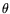for which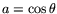and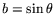.

Elements ofhave real components, but it is enlightening to consideras a subgroup of the group of all non-singular complex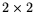matrices. Fact: any matrix inis similar to a matrix of the form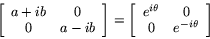Of course, the new basis vectors have complex components.

Example:, rotations in 3-space. Fact: any element ofleaves a line through the origin fixed. This seems obvious to anyone who has rolled a ball, but it is not totally trivial to prove. I will outline two arguments, both instructive.

First, an approach Euclid might have followed. Fact: any isometry of 3-space that leaves the origin fixed is the composition of at most three reflections. For consider the initial and final positions of the x, y, and z axes. One reflection moves the x-axis to its final position, pointing in the correct direction; a second reflection takes care of the y-axis; a third may be needed to reverse the direction of the z-axis. We see that an orientation-preserving isometry is the composition of two reflections. The intersection of the planes of these two reflections gives a line that is left fixed.

Next, a linear algebra approach. We want to show that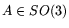leaves a vector fixed (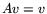), or in other words that 1 is an eigenvalue. Note first that the characteristic polynomial foris a cubic with real coefficients. Hence it has at least one real root. Furthermore,implies that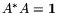trivially, since all components ofare real. By the spectral theorem,can be diagonalized (working over C) and its eigenvalues are all on the unit circle. Since the characteristic polynomial has real coefficients, non-real roots appear in conjugate pairs. It follows thatis similar to a matrix of the form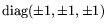or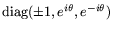. Since, and the determinant is the product of the roots, at least one eigenvalue must be 1.

The first argument can also be cast in linear algebra form. This leads to Householder transformations.Next: Lie Algebras Up: Lie Groups and Quantum Mechanics Previous: A Rough Road-map